JEE  >  JEE Advanced Level Test: Vector Algebra- 1

# JEE Advanced Level Test: Vector Algebra- 1 - JEE

Test Description

## 30 Questions MCQ Test Mathematics (Maths) for JEE Main & Advanced - JEE Advanced Level Test: Vector Algebra- 1

JEE Advanced Level Test: Vector Algebra- 1 for JEE 2023 is part of Mathematics (Maths) for JEE Main & Advanced preparation. The JEE Advanced Level Test: Vector Algebra- 1 questions and answers have been prepared according to the JEE exam syllabus.The JEE Advanced Level Test: Vector Algebra- 1 MCQs are made for JEE 2023 Exam. Find important definitions, questions, notes, meanings, examples, exercises, MCQs and online tests for JEE Advanced Level Test: Vector Algebra- 1 below.
 1 Crore+ students have signed up on EduRev. Have you?
JEE Advanced Level Test: Vector Algebra- 1 - Question 1

### The degree of the differential equation satisfying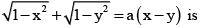Detailed Solution for JEE Advanced Level Test: Vector Algebra- 1 - Question 1

Putting x = sin A and y = sin B in the given relation, we get
cos A + cos B = a(sin A - sin B)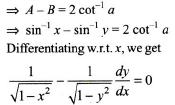Clearly, it is a differential equation of degree one

JEE Advanced Level Test: Vector Algebra- 1 - Question 2

### The differential equation whose solution is Ax2 + By2 = 1, where A and B are arbitrary constants, is of

Detailed Solution for JEE Advanced Level Test: Vector Algebra- 1 - Question 2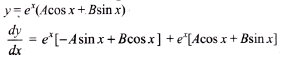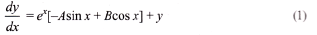Again differentiating w.r.t. x, we get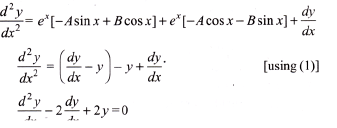JEE Advanced Level Test: Vector Algebra- 1 - Question 3

### Differential equation of the family of circles touching the line y = 2 at (0, 2) is

Detailed Solution for JEE Advanced Level Test: Vector Algebra- 1 - Question 3

Options A, B, C – all have a family of circles with centre (0,2).
But according to given conditions (0,2) is a point on the circle.
So, none of the options are correct.

JEE Advanced Level Test: Vector Algebra- 1 - Question 4

The differential equation of all parabolas whose axis are parallel to the y-axis is

Detailed Solution for JEE Advanced Level Test: Vector Algebra- 1 - Question 4

The equation of a member of the family of parabolas having axis parallel toy-axis is
y — Ax2 + Bx+ C     ......(1)
where A, B, and C are arbitrary constants
Differentiating equation (1) w.r.t. x, we get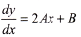......(2)
which on again differentiating w.r.t, x gives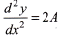....(3)
Differentiating (3) w.r.t. x, we get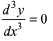JEE Advanced Level Test: Vector Algebra- 1 - Question 5

The differential equation of all circles which pass through the origin and whose centers lie on the y-axis is

Detailed Solution for JEE Advanced Level Test: Vector Algebra- 1 - Question 5

If (0, k) be the centre ony-axis then its radius will be k as it passes through origin. Hence its equation is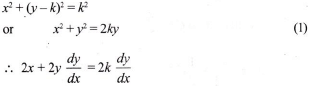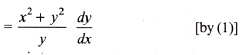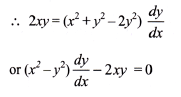JEE Advanced Level Test: Vector Algebra- 1 - Question 6

The differential equation whose general solution is given by, y =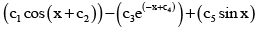, where c1, c2, c3, c4, c5 are arbitrary constants, is

Detailed Solution for JEE Advanced Level Test: Vector Algebra- 1 - Question 6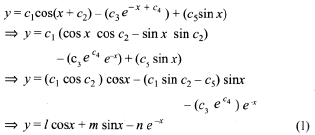where l, m, n are arbitrary constant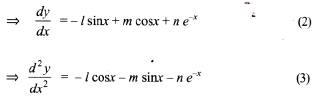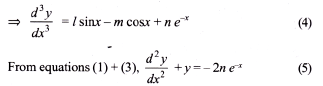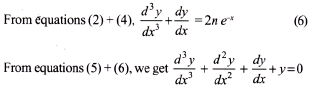JEE Advanced Level Test: Vector Algebra- 1 - Question 7

The equation of the curves through the point (1, 0) and whose slope is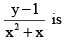Detailed Solution for JEE Advanced Level Test: Vector Algebra- 1 - Question 7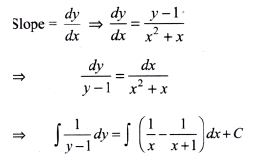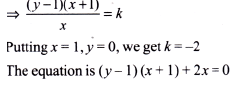JEE Advanced Level Test: Vector Algebra- 1 - Question 8

The solution of the equation log(dy/dx) = ax + by is

Detailed Solution for JEE Advanced Level Test: Vector Algebra- 1 - Question 8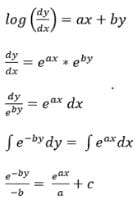JEE Advanced Level Test: Vector Algebra- 1 - Question 9

Solution of differential equation dy – sin x sin ydx = 0 is

Detailed Solution for JEE Advanced Level Test: Vector Algebra- 1 - Question 9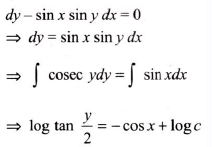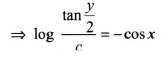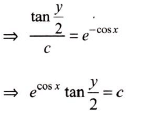JEE Advanced Level Test: Vector Algebra- 1 - Question 10

The solution of the equation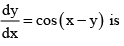Detailed Solution for JEE Advanced Level Test: Vector Algebra- 1 - Question 10

Putting u = x - y, we get du/dx = 1 - dy/dx. The given equation can be written as 1 - du/dx = cos u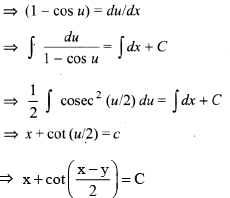JEE Advanced Level Test: Vector Algebra- 1 - Question 11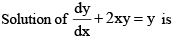Detailed Solution for JEE Advanced Level Test: Vector Algebra- 1 - Question 11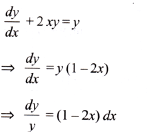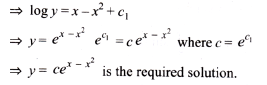JEE Advanced Level Test: Vector Algebra- 1 - Question 12

The general solution of the differential equation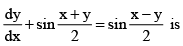Detailed Solution for JEE Advanced Level Test: Vector Algebra- 1 - Question 12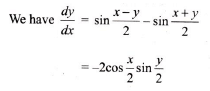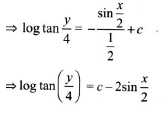JEE Advanced Level Test: Vector Algebra- 1 - Question 13

The solutions of (x + y + 1) dy = dx is

Detailed Solution for JEE Advanced Level Test: Vector Algebra- 1 - Question 13

Putting x + y + 1 = u, we have du = dx + dy and the given equation reduces to u(du - dx) = dx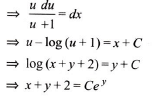JEE Advanced Level Test: Vector Algebra- 1 - Question 14

The slope of the tangent at (x, y) to a curve passing through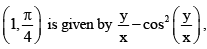then the equation of the curve is

Detailed Solution for JEE Advanced Level Test: Vector Algebra- 1 - Question 14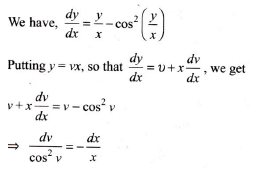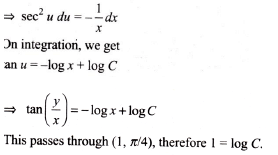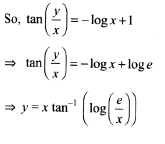JEE Advanced Level Test: Vector Algebra- 1 - Question 15

The solution of (x2 + xy)dy = (x2 + y2)dx is

Detailed Solution for JEE Advanced Level Test: Vector Algebra- 1 - Question 15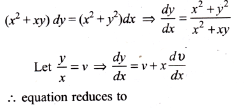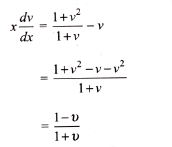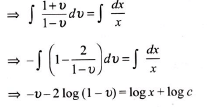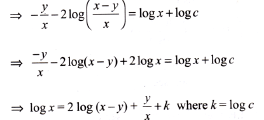JEE Advanced Level Test: Vector Algebra- 1 - Question 16

The solution of (y + x + 5)dy = (y – x + 1) dx is

Detailed Solution for JEE Advanced Level Test: Vector Algebra- 1 - Question 16

The intersection of y – x + 1 = 0 and y + x + 5 = 0 is (-2, -3).
Put x = X - 2, y = Y - 3
The given equation reduces to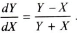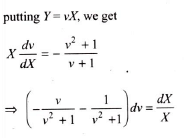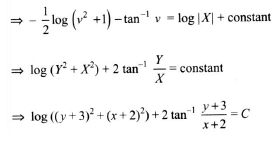JEE Advanced Level Test: Vector Algebra- 1 - Question 17

The slope of the tangent at (x, y) to a curve passing through a point (2, 1) is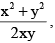then the equation of the curve is

Detailed Solution for JEE Advanced Level Test: Vector Algebra- 1 - Question 17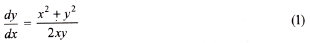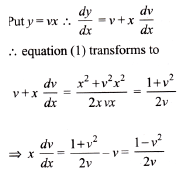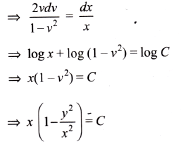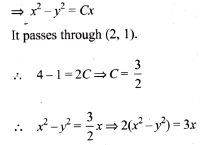JEE Advanced Level Test: Vector Algebra- 1 - Question 18

The solution of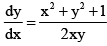satisfying y(1) = 1 is given by

Detailed Solution for JEE Advanced Level Test: Vector Algebra- 1 - Question 18

Rewritting the given equation as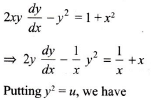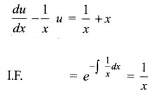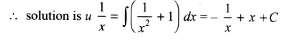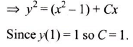Hence y2 = x(1 + x) - 1 which represents a system of hyperbola.

JEE Advanced Level Test: Vector Algebra- 1 - Question 19

Solution of the equation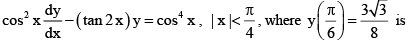Detailed Solution for JEE Advanced Level Test: Vector Algebra- 1 - Question 19

The given differential equation can be written as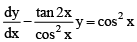which is linear differential equation of first order.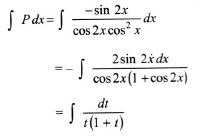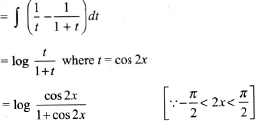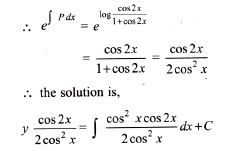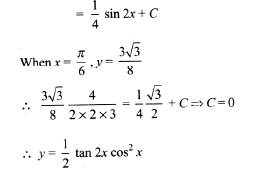JEE Advanced Level Test: Vector Algebra- 1 - Question 20

A function y = f(x) satisfies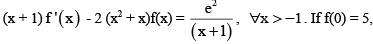then f(x) is

Detailed Solution for JEE Advanced Level Test: Vector Algebra- 1 - Question 20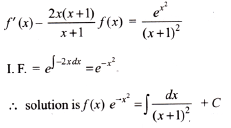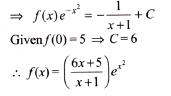JEE Advanced Level Test: Vector Algebra- 1 - Question 21

The general solution of the equation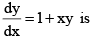Detailed Solution for JEE Advanced Level Test: Vector Algebra- 1 - Question 21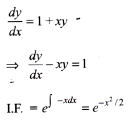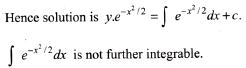JEE Advanced Level Test: Vector Algebra- 1 - Question 22

The curve satisfying the equation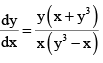and passing through the point (4, - 2) is

Detailed Solution for JEE Advanced Level Test: Vector Algebra- 1 - Question 22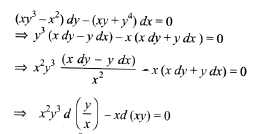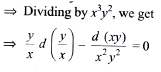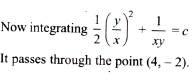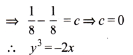JEE Advanced Level Test: Vector Algebra- 1 - Question 23

The solution of the differential equation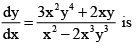Detailed Solution for JEE Advanced Level Test: Vector Algebra- 1 - Question 23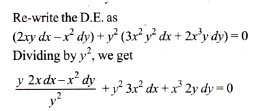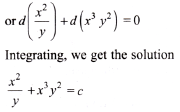JEE Advanced Level Test: Vector Algebra- 1 - Question 24

Which of the following is not the differential equation of family of curves whose tangent from an angle of π/4 with the hyperbola xy = c2

Detailed Solution for JEE Advanced Level Test: Vector Algebra- 1 - Question 24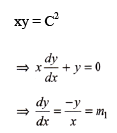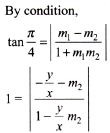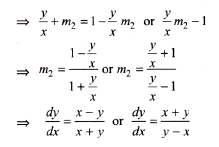JEE Advanced Level Test: Vector Algebra- 1 - Question 25

Tangent to a curve intercepts the y-axis at a point P. A line perpendicular to this tangent through P passes through another point (1, 0). The differential equation of the curve is

Detailed Solution for JEE Advanced Level Test: Vector Algebra- 1 - Question 25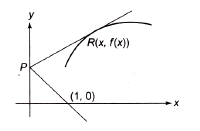The equation of the tangent at the point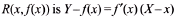The coordinates of the point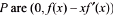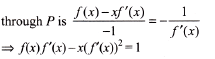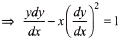which is the required differential equation to the curve at y =f(x).

JEE Advanced Level Test: Vector Algebra- 1 - Question 26

The curve for which the normal at any point (x, y) and the line joining the origin to that point from an isosceles triangle with the x-axis as base is

Detailed Solution for JEE Advanced Level Test: Vector Algebra- 1 - Question 26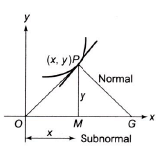t is given that the triangle OPC is an isosceles triangle.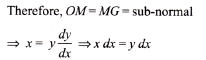On integration, we get x2-y2 = C, which is a rectangular hyperbola.

JEE Advanced Level Test: Vector Algebra- 1 - Question 27

A normal at P(x, y) on a curve meets the x-axis at Q and N is the foot of the ordinate at P. If NQ =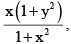then the equation of curve given that it passes through the point (3,1) is

Detailed Solution for JEE Advanced Level Test: Vector Algebra- 1 - Question 27

Let the equation of the  curve be y = f (x).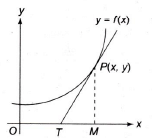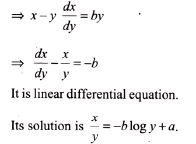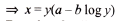JEE Advanced Level Test: Vector Algebra- 1 - Question 28

The equation of the curve passing through (2, 7/2) and having gradient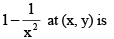Detailed Solution for JEE Advanced Level Test: Vector Algebra- 1 - Question 28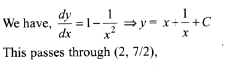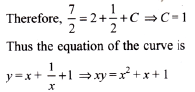JEE Advanced Level Test: Vector Algebra- 1 - Question 29

A normal at any point (x, y) to the curve y = f(x) cuts a triangle of unit area with the axis, the differential equation of the curve is

Detailed Solution for JEE Advanced Level Test: Vector Algebra- 1 - Question 29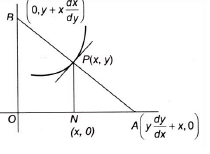Equation of normal at point p is
Y – y = (dx/dy)(X – x)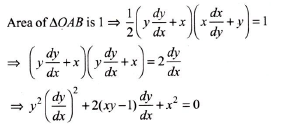JEE Advanced Level Test: Vector Algebra- 1 - Question 30

The differential equation of all parabola each of which has a latus rectum 4a and whose axis parallel to the x-axis is

Detailed Solution for JEE Advanced Level Test: Vector Algebra- 1 - Question 30

Equation to the family of parabolas is (y - k)2 = 4a(x - h).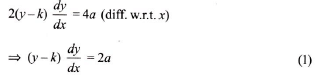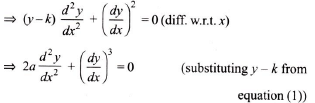Hence the order is 2 and the degree is 1.

## Mathematics (Maths) for JEE Main & Advanced

129 videos|408 docs|306 tests